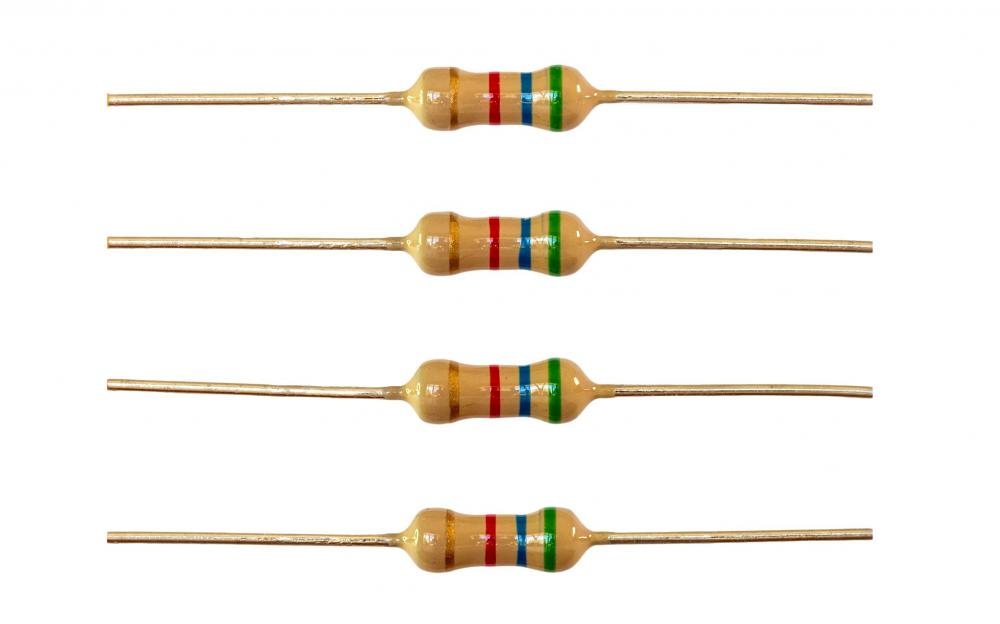# What is a Fixed Resistor?

Carol Francois

A fixed resistor is part of an electric circuit and is used to reduce the flow of electricity. Resistance is measured in Ohms and is typically shown as the number and then the units; for example, a 750 Ohm resistor would be written as 750-Ohm. The size of the resistor is based on the number of Ohms, and it can range from pin to book size.A fixed resistor reduces the flow of electricity within an electrical circuit.

Resistors come in two classes: fixed and variable. A fixed resistor is set at a specific value and cannot be changed. A variable resistor is able to manage flows at a specific level and below. This is an important distinction and determines when and where a resistor should be used.A variable resistor is able to manage flows of electricity at a specific level as well as below that level.

When selecting a resistor, it is important for a person to consider three things: the value of the resistor, its tolerance, and its power rating. The value is measured in Ohms. The tolerance indicates the upper and lower bounds of actual performance, which is measured in plus and minus percentage. For example, a tolerance of 10% means that the resistor performs within a 10% range of the resistance value listed in the specifications.

Power rating shows the upper limit of power that can be managed by the resistor and is measured in watts. To calculate the power, a person can multiply the resistance value of the resistor by the square root of the current. If the power rating is exceeded, the resistor will fail. A rule of thumb is to use a resistor with a power rating two times higher than the actual power needed.

There are two kinds of fixed resistors: carbon and metal film. Carbon film resistors are designed for general use and are fairly cheap to produce and purchase. These units have a tolerance of 5%, with power ratings of 1/8 Watts (W), 1/4W, and 1/2W. The primary issue with this type of resistor is the fact they generate electrical noise.

A metal film resistor is best used when a higher tolerance is required. These units have a greater level of accuracy than carbon film resistors, due to the nature of the materials used. There is a corresponding increase in price, but it may be well worth the incremental cost to protect the other components of the circuitry.

When reviewing the different types of resistors, individuals should think about the intended use of the circuit. They should select a manufacturer with a good reputation for quality and consistency, and take the time to test the resistor and the circuit before installation to ensure all the specifications are correct.A resistor is an electronic component that can lower a circuit’s voltage and its flow of electrical current.

## You might also Likeanon270033

What's a variable resistor?

allenJo

@NathanG - Even after you build the circuit, I think you can still test the resistance values. I used to have something called a multimeter which I could use to test resistance levels at different points in the circuit.

It was very accurate and would let me know if the resistor was working properly. Of course, if the readings I got didn’t match the rating on the resistor, that usually meant that the resistor had been blown out or something was wrong somewhere else in the circuit.

NathanG

I used to buy resistors in bulk when I built electronic circuits as a hobby. Most of the packages I bought were from the local electronics supply store, and as I was mostly following schematic diagrams in books, the resistance, ohms and other ratings were supplied to me. Rarely did I need to figure anything out.

However, sometimes I would branch out on my own, building circuits from scratch, in which case I did need to come up with the correct numbers.

One thing that I found which helped me was the resistor calculator. This was an online application which asked me to enter the voltage and desired current. It then gave me the ohms and watts as an output so I could pick the right resistor rating for my project.

After awhile it got to the point where I could estimate what the correct ratings would be.